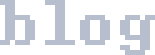skip nav

### Calculating a Bounce

Problem: Given a ball and a curved wall, how do we calculate the angle of the bounce of the ball? Assuming we have the normal of the wall at the bounce location, our problem becomes:

Problem: Given two vectors, x₁ and n, how do we mirror vector x₁ around vector n to get x′? (x₁ is the ball velocity and n is the normal of the wall.)

Solution: Implement https://mathworld.wolfram.com/Reflection.html (The first picture is accurate to the situation.)

As written: x₁′ = -x₁ + 2x₀ + 2n̂[(x₁-x₀)·n̂]

Given that x₀ is always [0,0], it can be ignored.

x₁′ = -x₁ + 2n̂[x₁·n̂]

Given that n is pre-normalized, we can un-hat the ns.

x₁′ = -x₁ + 2n[x₁·n]

To calculate the dot product: (from https://www.mathsisfun.com/algebra/vectors-dot-product.html)

x₁′ = -x₁ + 2n[x₁*n+x₁*n]

Normalize the notation, since we're now using  to get the vector components.

x₁′ = -x₁ + 2*n*(x₁*n+x₁*n)

Now, to calculate both parts of the vector separately:

x₁′ = -x₁ + 2*n*(x₁*n+x₁*n)

x₁′ = -x₁ + 2*n*(x₁*n+x₁*n)

Now you can replace the x₁ and n with the variables of your program, and be on your way. For example, in Javascript:

`//Returns vector v mirrored around the normalized vector mir.` `function vReflectIn(v,mir) { ` ` return [` ` -v + 2*mir*(v*mir+v*mir),` ` -v + 2*mir*(v*mir+v*mir),` ` ]; ` `}`